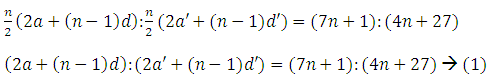##### Get a free home demo of LearnNext

Available for CBSE, ICSE and State Board syllabus.
Call our LearnNext Expert on 1800 419 1234 (tollfree)
OR submit details below for a call back

clear

Venkatesh

Nov 13, 2013

# The ratio of the sum of n terms of two A.P.s is (7n+1):(4n+27).

The ratio of the sum of n terms of two A.P.s is (7n+1):(4n+27).Find the ratio of their mth term.

Syeda

Answer. Given ratio of sum of n terms of two AP’s = (7n+1):(4n+27) Let’s consider the ratio these two AP’s mth terms as am : a’m →(2) Recall the nth term of AP formula, an = a + (n – 1)d Hence equation (2) becomes, am : a’m = a + (m – 1)d : a’ + (m – 1)d’ On multiplying by 2, we get am : a’m = [2a + 2(m – 1)d] : [2a’ + 2(m – 1)d’] = [2a + {(2m – 1) – 1}d] : [2a’ + {(2m – 1) – 1}d’] = S2m – 1 : S’2m – 1 = [7(2m – 1) + 1] : [4(2m – 1) +27] [from (1)] = [14m – 7 +1] : [8m – 4 + 27] = [14m – 6] : [8m + 23] Thus the ratio of mth terms of two AP’s is [14m – 6] : [8m + 23].

Agam Gupta

Given ratio of sum of n terms of two AP’s = (7n+1):(4n+27)Let’s consider the ratio these two AP’s mth terms as am : a’m →(2) Recall the nth term of AP formula, an = a + (n – 1)d Hence equation (2) becomes, am : a’m = a + (m – 1)d : a’ + (m – 1)d’ On multiplying by 2, we get am : a’m = [2a + 2(m – 1)d] : [2a’ + 2(m – 1)d’] = [2a + {(2m – 1) – 1}d] : [2a’ + {(2m – 1) – 1}d’] = S2m – 1 : S’2m – 1  = [7(2m – 1) + 1] : [4(2m – 1) +27] [from (1)] = [14m – 7 +1] : [8m – 4 + 27] = [14m – 6] : [8m + 23] Thus the ratio of mth terms of two AP’s is [14m – 6] : [8m + 23].

Dinesh Singha

Given ratio of sum of n terms of two AP’s = (7n+1):(4n+27)Let’s consider the ratio these two AP’s mth terms as am : a’m →(2) Recall the nth term of AP formula, an = a + (n – 1)d Hence equation (2) becomes, am : a’m = a + (m – 1)d : a’ + (m – 1)d’ On multiplying by 2, we get am : a’m = [2a + 2(m – 1)d] : [2a’ + 2(m – 1)d’] = [2a + {(2m – 1) – 1}d] : [2a’ + {(2m – 1) – 1}d’] = S2m – 1 : S’2m – 1  = [7(2m – 1) + 1] : [4(2m – 1) +27] [from (1)] = [14m – 7 +1] : [8m – 4 + 27] = [14m – 6] : [8m + 23] Thus the ratio of mth terms of two AP’s is [14m – 6] : [8m + 23].

Priyanshu

Given ratio of sum of n terms of two AP’s = (7n+1):(4n+27)Let’s consider the ratio these two AP’s mth terms as am : a’m →(2) Recall the nth term of AP formula, an = a + (n – 1)d Hence equation (2) becomes, am : a’m = a + (m – 1)d : a’ + (m – 1)d’ On multiplying by 2, we get am : a’m = [2a + 2(m – 1)d] : [2a’ + 2(m – 1)d’] = [2a + {(2m – 1) – 1}d] : [2a’ + {(2m – 1) – 1}d’] = S2m – 1 : S’2m – 1 = [7(2m – 1) + 1] : [4(2m – 1) +27] [from (1)] = [14m – 7 +1] : [8m – 4 + 27] = [14m – 6] : [8m + 23] Thus the ratio of mth terms of two AP’s is [14m – 6] : [8m + 23].

Kishore Kumar

Given ratio of sum of n terms of two AP’s = (7n+1):(4n+27)Let’s consider the ratio these two AP’s mth terms as am : a’m →(2) Recall the nth term of AP formula, an = a + (n – 1)d Hence equation (2) becomes, am : a’m = a + (m – 1)d : a’ + (m – 1)d’ On multiplying by 2, we get am : a’m = [2a + 2(m – 1)d] : [2a’ + 2(m – 1)d’] = [2a + {(2m – 1) – 1}d] : [2a’ + {(2m – 1) – 1}d’] = S2m – 1 : S’2m – 1 = [7(2m – 1) + 1] : [4(2m – 1) +27] [from (1)] = [14m – 7 +1] : [8m – 4 + 27] = [14m – 6] : [8m + 23] Thus the ratio of mth terms of two AP’s is [14m – 6] : [8m + 23].

STAFF-95393


###### Like NextGurukul? Also explore our advanced self-learning solution LearnNext
Offered for classes 6-12, LearnNext is a popular self-learning solution for students who strive for excellence
Explore
Animated Video
lessons
All India
Test Series
Interactive Video
Experiments
Best-in class
books